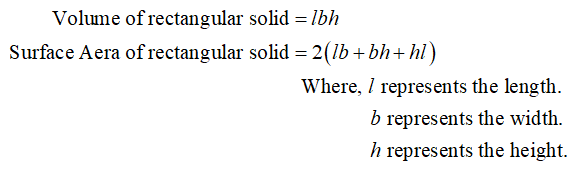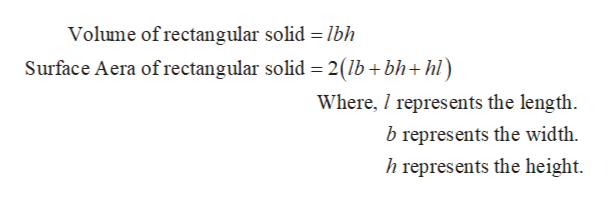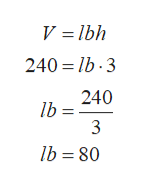# A closed box is in the shape of a rectangular solid with height 3 m. Its surface area is 268 m2. If the volume is 240 m3, find the dimensions of the box.

Question
7 views

A closed box is in the shape of a rectangular solid with height 3 m. Its surface area is 268 m2. If the volume is 240 m3, find the dimensions of the box.

check_circle

Step 1

Consider the closed box is in the shape of rectangular solid having height 3 m. The surface area of rectangular box is 268 m2 and volume is 240 m3.

Step 2

The formula of surface area and volume of rectangular solid.help_outlineImage TranscriptioncloseVolume of rectangular solid = lbh Surface Aera of rectangular solid = 2(lb+bh+hl) Where, I represents the length. b represents the width. h represents the height. fullscreen
Step 3

The volume of rectang...help_outlineImage TranscriptioncloseV = lbh 240 = lb · 3 240 Ib = 3 lb = 80 fullscreen

### Want to see the full answer?

See Solution

#### Want to see this answer and more?

Solutions are written by subject experts who are available 24/7. Questions are typically answered within 1 hour.*

See Solution
*Response times may vary by subject and question.
Tagged in